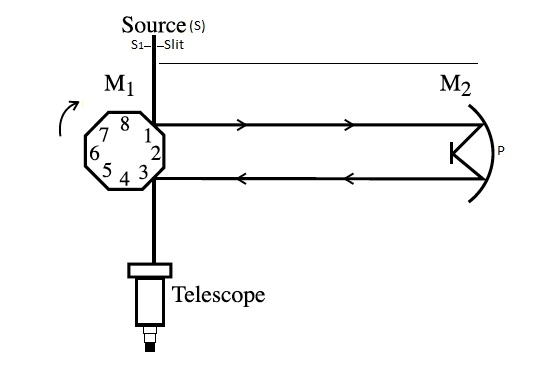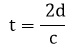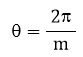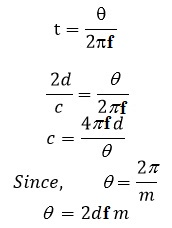# Velocity of Light Michelsons’s Method

Michelsons’s Method is a precise method for measuring the speed of light. An octagonal mirror M1 is mounted on the shaft of a variable speed motor. Light from a bright source S is focused at an angle of 45o on one of the faces of mirror M1 after passing through a slit S1. The reflected light falls on a distant concave mirror M2. In figure, M3 is a plane mirror and with the help of this mirror M3 placed at the center of curvature of mirror M2 the beam of light is returned back and falls on face 3 of the octagonal mirror M1 again at an angle of 45o. The light reflected by this face is then collected by a telescope T and the eye of the telescope.

If the mirror M1 is rotated, the light returning to it from the mirror M2 will not be incident at an angle of 45o, and hence will not enter the telescope. When the speed of rotation of the mirror M1 is so adjusted that the face 2 of mirror occupies exactly the same position as was occupied by face 3 earlier (in 1/8th revolution, of mirror M1) during hte time light travels from M1 to M2 and back to M1, then the image of source will reappear.Fig – Michealson’s method for the determination of velocity of light

If d be the distance between the mirror M1 and M2 and c be the speed of light, then the time taken by the light to travel from M1 to M2 and back to M1 isIf f is the number of revolutions per seconds of mirror M1 and m is the number of faces of this mirror, then the angle rotated by the mirror during the time t isNow,The speed of rotation of mirror M1 can be measured by using stroboscope. In Michelson’s original experiment, the speed of the motor was about 500 rev/s and d was about 35 km. he obtained a value of 2.99775 x 108 ms-1 for speed of light in vacuum.

The modern methods of measuring speed of light use coherent laser beams and radar signals and give results with high degree of accuracy. The most probable value for the speed of light in free space is c = 2.99792458 x 108 ms-1 .